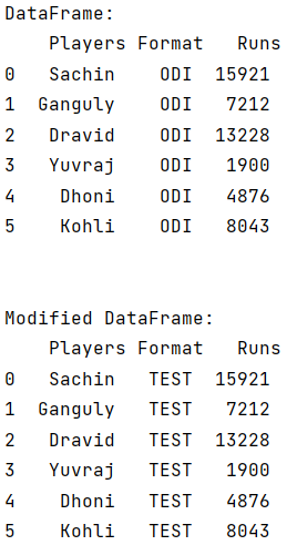# Pandas: How to replace all values in a column, based on condition?

Given a Pandas DataFrame, we have to replace all values in a column, based on the given condition.
Submitted by Pranit Sharma, on May 29, 2022

Columns are the different fields that contain their particular values when we create a DataFrame. We can perform certain operations on both rows & column values.

By replacing all the values based on a condition, we mean changing the value of a column when a specific condition is satisfied.

This task can be done in multiple ways, we will use pandas.DataFrame.loc property to apply a condition and change the value when the condition is true.

To work with pandas, we need to import pandas package first, below is the syntax:

```import pandas as pd
```

Let us understand with the help of an example.

```# Importing pandas package
import pandas as pd

# creating a dictionary of student marks
d = {
"Players":['Sachin','Ganguly','Dravid','Yuvraj','Dhoni','Kohli'],
"Format":['ODI','ODI','ODI','ODI','ODI','ODI'],
"Runs":[15921,7212,13228,1900,4876,8043]
}

# Now we will create DataFrame
df = pd.DataFrame(d)

# Viewing the DataFrame
print("DataFrame:\n",df,"\n\n")

# Replacing thr values of column Format
df.loc[(df.Format == 'ODI' ), 'Format'] = 'TEST'

# Display modified DataFrame
print("Modified DataFrame:\n",df)
```

Output:Languages: » C » C++ » C++ STL » Java » Data Structure » C#.Net » Android » Kotlin » SQL
Web Technologies: » PHP » Python » JavaScript » CSS » Ajax » Node.js » Web programming/HTML
Solved programs: » C » C++ » DS » Java » C#
Aptitude que. & ans.: » C » C++ » Java » DBMS
Interview que. & ans.: » C » Embedded C » Java » SEO » HR
CS Subjects: » CS Basics » O.S. » Networks » DBMS » Embedded Systems » Cloud Computing
» Machine learning » CS Organizations » Linux » DOS
More: » Articles » Puzzles » News/Updates